# On picking an orthogonal vector (and combing coconuts)

Consider the following problem:

Given a non-zero vector v in 3D space, find a non-zero vector w orthogonal to v (i.e. such that v.w = 0).

At first sight, this seems easy. For every possible v there are an infinite number of orthogonal vectors lying in a plane:We just need to pick one. But it’s not as trivial as it may seem.

## First attempts

The following vector satisfies v.w1 = 0:Unfortunately that w1 vector can be zero, for instance when v = [0 0 1]. The original problem requires a non-zero vector.

Let’s try another one: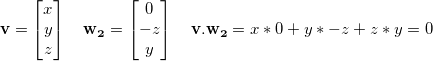Again, it works most of the time, except in some cases, for instance v = [1 0 0].

How about adding them together? Certainly w1 and w2 cannot be both zero at the same time!Alas, this still doesn’t work all the time: it fails with v = ![1 0 1].

Should we try harder? Isn’t there a linear combination of these that will work all the time?

Well, no. There isn’t.

## You can’t comb a coconut

Sadly, the hairy ball theorem, a consequence of Brouwer’s fixed-point theorem, states that any continuous function f that maps the set of unit vectors to orthogonal vectors takes the value zero somewhere.

Whichever way you look at the sphere of unit vectors, a continuous tangent field will always have a zero point:Most of the maths operations we usually do (adding, multiplying, taking square roots, etc.) are continuous. Fortunately, we are not limited to them: we can use a simple if() construct to create a discontinuity that will save us.

## A very good implementation

Here is my personal implementation of the orthogonal vector problem. It is very good. It has excellent numerical stability. It only does one test. It is short. It is beautiful. I really hope you consider using it.

/* Always works if the input is non-zero.
* Doesn’t require the input to be normalised.
* Doesn’t normalise the output. */
vec3 orthogonal(vec3 v)
{
return abs(v.x) > abs(v.z) ? vec3(-v.y, v.x, 0.0)
: vec3(0.0, -v.z, v.y);
}


Many implementations, such as Ogre3D’s, have additional tests or operations to perform this task, and use epsilons and normalised vectors to avoid singularities.

But our only test is actually enough: if |x|>|z|, it means the largest component in absolute value in v is either x or y. So we build a vector using x and y. Otherwise, the largest component in absolute value is either y or z. So we build a vector using y and z. That way, we ensure that the length of our returned vector never, ever comes near zero.

Whether some given code will cause inefficient branching is often unclear. In our case, it is very likely that the ternary operator will actually be branchless, with some help of the compiler and the hardware.

That said, how about we try to get rid of the ternary operator, just for fun?

## Going branchless for fun

Let’s see. We had these two candidate vectors, w1 and w2, which worked almost always except when specific values of v caused them to be zero. And whatever the constant k we may pick, a vector of the form w1 + k w2 will eventually take the value zero for some value of v, too, because of the hairy ball theorem.

Now here comes the trick. Instead of using a constant k, we use a function f(x,y,z). This is what our w vector looks like: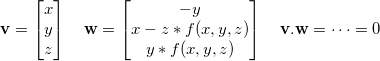From now I shall assume that v is a unit vector.

What can cause w to be zero, then?

One necessary condition is y = 0. When y ≠ 0 we can chose anything we want for f(x,y,z), it’ll always give us a good orthogonal vector. This restricts the problem to the y = 0 circle, giving us the useful equality x² + z² = 1.

The other condition is x = z*f(x,y,z). So if we manage to build a function f such that f(x,y,z) never equals x/z, we win.

Using x² + z² = 1 we can plot all the possible values for x/z as a function of x. It will show us, for a given x, the values that f cannot take:The almost vertical slopes you see go to infinity upwards and downwards. As expected, this prevents us from using a continuous function: it would obviously cross one of these walls at some point.

Well, let’s try a non-continuous function, then. What are our options?

• fmod
• floor, ceil, round
• fract

Here is one that I came up with and which works reasonably well: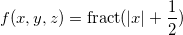Look how it nicely avoids x/z:And finally, our resulting branchless code:

/* Requires the input to be normalised.
* Doesn’t normalise the output. */
vec3 orthogonal(vec3 v)
{
float k = fract(abs(v.x) + 0.5);
return vec3(-v.y, v.x - k * v.z, k * v.y);
}


I find it highly unlikely that this second version will perform better than the branching one. However, I haven’t put much thought into it and maybe someone will come up with a much better solution using the ideas presented here. Have fun!

# L’histoire du t-shirt Casimir

Il y a bien longtemps, m’inspirant d’un de mes dessinateurs préférés, j’ai commis ce dessin idiot avec l’idée d’en faire un t-shirt :Je l’ai donc uploadé sur la plateforme Comboutique. Malheureusement, en l’espace de quelques heures il était supprimé, avec cette justification :

Bonjour,

nous sommes contraint d'effacer votre visuel :

casimir-blue.png

qui est en infraction avec le code de la propriété intellectuelle
(industrielle, commerciale, littéraire et artisitique) ou qui est d'une
résolution insuffisante pour pouvoir être sérigraphié sur un t-shirt.
Nous vous demandons de bien veiller à être absolument certains de
disposer des droits sur un visuel avant de l'uploader sur le serveur
Comboutique.

Autant dire que je me suis senti blessé autant en droit qu’en grammaire. Visiblement ma réponse a fait marrer tout le monde, la voici donc :

From: Sam Hocevar <sam@zoy.org>
Date: Tue, 24 Jan 2006 18:36:00 +0100
To: service.client@comboutique.com
Subject: Re: Comboutique.com - Suppression d'un visuel délictueux

On Tue, Jan 24, 2006, comboutique.com wrote:

>    Bonjour,

Bonjour !

>    nous sommes contraint d'effacer votre visuel :
>
>    casimir-blue.png
>
>    qui est en infraction avec le code de la propriété intellectuelle
>    (industrielle, commerciale, littéraire et artisitique) ou qui est d'une
>    résolution insuffisante pour pouvoir être sérigraphié sur un t-shirt.

Je me demande quelle interprétation vous pouvez bien avoir du code
de la propriété intellectuelle pour décider qu'il m'est impossible de
pasticher Casimir, sachant que l'auteur lui-même d'une oeuvre ne peut
s'opposer au pastiche (article L122-5). Mais je suppose que je ne vous
apprends rien, puisque pendant plusieurs mois début 2005  parmi vos
meilleures ventes figuraient des pastiches de l'oeuvre de feu Roger
Hargreaves, et sans l'exception de pastiche, Chorion PLC (qui gère aussi
les droits d'Agatha Christie et Georges Simenon, autant dire que leur
sens de l'humour doit valoir celui d'une pelleteuse) vous aurait sans
doute taillé un short à la flamme bien moyenâgeuse.

D'ailleurs avec des boutiques comme HOT-COUTURE  on peut dire
que vous tenez à être au top de la recherche en droit, puisque vous
vous basez sur la jurisprudence "Esso contre Greenpeace" de 2003 qui
transfère l'exception de parodie au droit des marques (ce qui est quand
même un petit peu fort de café, en tout cas moi je n'aurais pas osé).

Et je suis bien conscient que la dérogation de parodie suppose « un
travail de travestissement ou de subversion de l'oeuvre parodiée, que
le public perçoit comme tel » (jurisprudences "Tintin" et "Caliméro"
entre autres), aussi ai-je fait mon possible pour qu'aucun doute ne soit
possible de la part du public (puisqu'on en parle, je tiens à préciser
que j'ai aussi fait tout mon possible pour ne pas dépasser en coloriant,
ce qui méritait d'être dit).

Si votre service juridique considère que pourvoir Casimir d'un sexe
massif et richement innervé ne constitue pas un acte subversif suffisant
pour établir l'exception de parodie, je suis ouvert à toute suggestion
allant dans ce sens : je pense notamment à une pilosité plus fournie et
une érection démesurée, mais peut-être avez-vous de meilleures idées. Si
vous préférez que Casimir mange son caca et vomisse sur des enfants, je
suis désolé mais vous allez trop loin.

>    Nous vous demandons de bien veiller à être absolument certains de
>    disposer des droits sur un visuel avant de l'uploader sur le serveur
>    Comboutique.

Ben excusez-moi mais c'est quand même vous qui me l'avez effacé avant
que je ne puisse dire quoi que ce soit. Vous n'êtes vraiment pas très
sympa.

Très cordialement,
--
Sam. <http://sam.zoy.org/>

1. http://web.archive.org/web/20050206182446/www.comboutique.com/shop/homepage.php
2. http://www.comboutique.com/hotcouture

# Beautiful maths simplification: quaternion from two vectors

In this article I would like to explain the thought process behind the derivation of a widely used formula: finding a quaternion representing the rotation between two 3D vectors. Nothing really new, but hopefully a few ideas could be reused at other times.

Note: the routine presented here is incomplete on purpose. For a version that can be used in production code, see the next article instead, Quaternion from two vectors: the final version.

## Naive method

A rotation is best visualised using a rotation axis and an angle. Except in degenerate cases, the rotation axis can be obtained by computing the cross product of the two original vectors:Then the angle can be obtained using the properties of the cross product and/or the dot product:Since θ is always between 0 and π, we only care about the dot product. This gives us some obvious code to create the quaternion (omitting corner cases such as θ = 0 for clarity):

quat quat::fromtwovectors(vec3 u, vec3 v)
{
float cos_theta = dot(normalize(u), normalize(v));
float angle = acos(cos_theta);
vec3 w = normalize(cross(u, v));
return quat::fromaxisangle(angle, w);
}


This is naive but it works. Googling for “quaternion from two vectors” shows this forum post and this SO question where this method or a variation thereof is suggested.

## Looking under the hood

Let’s have a look at what happens when building the quaternion from an axis and an angle: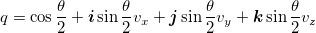This means the code for quat::fromaxisangle would look somewhat like this:

quat quat::fromaxisangle(float angle, vec3 axis)
{
float half_sin = sin(0.5f * angle);
float half_cos = cos(0.5f * angle);
return quat(half_cos,
half_sin * axis.x,
half_sin * axis.y,
half_sin * axis.z);
}


## Avoiding trigonometry

If you read Iñigo Quilez’s recent article about avoiding trigonometry you’ll have probably frowned at the fact that we computed θ from cos(θ), then computed sin(θ/2) and cos(θ/2).

Indeed, it happens that there is a much simpler way to do it; the half-angle formulas from precalculus tell us the following: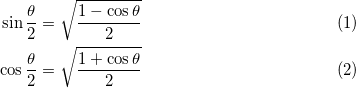This allows us to simplify our quaternion creation code:

quat quat::fromtwovectors(vec3 u, vec3 v)
{
float cos_theta = dot(normalize(u), normalize(v));
float half_cos = sqrt(0.5f * (1.f + cos_theta));
float half_sin = sqrt(0.5f * (1.f - cos_theta));
vec3 w = normalize(cross(u, v));
return quat(half_cos,
half_sin * w.x,
half_sin * w.y,
half_sin * w.z);
}


This is pretty nice. By using well known trigonometry formulas, we got rid of all trigonometry function calls!

## Avoiding square roots

It happens that we can do slightly better. Note that we normalize three vectors: u, v and cross(u, v). That’s three square roots. The thing is, we already know the norm of w through this formula: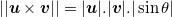And we know sin(θ) from precalculus again: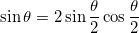Also, using the fact that sqrt(a)sqrt(b) = sqrt(ab) lets us perform one less square root.

We can therefore come up with the following performance improvement:

quat quat::fromtwovectors(vec3 u, vec3 v)
{
float norm_u_norm_v = sqrt(sqlength(u) * sqlength(v));
float cos_theta = dot(u, v) / norm_u_norm_v;
float half_cos = sqrt(0.5f * (1.f + cos_theta));
float half_sin = sqrt(0.5f * (1.f - cos_theta));
vec3 w = cross(u, v) / (norm_u_norm_v * 2.f * half_sin * half_cos);
return quat(half_cos,
half_sin * w.x,
half_sin * w.y,
half_sin * w.z);
}


Oh wait! We divide by sin(θ/2) to compute w, then we multiply by sin(θ/2) again. This means we don’t even need that variable, and we can simplify even further:

quat quat::fromtwovectors(vec3 u, vec3 v)
{
float norm_u_norm_v = sqrt(sqlength(u) * sqlength(v));
float cos_theta = dot(u, v) / norm_u_norm_v;
float half_cos = sqrt(0.5f * (1.f + cos_theta));
vec3 w = cross(u, v) / (norm_u_norm_v * 2.f * half_cos);
return quat(half_cos, w.x, w.y, w.z);
}


This is more or less the code used by the Ogre3D engine in OgreVector3.h, except they perform an additional normalisation step on the final result. This is mathematically useless, but due to numerical stability issues, it is probably safe to do so nonetheless.

This final normalisation step is actually an opportunity to simplify the code even further.

## Improving on Ogre3D

We are down to two square roots and four divisions, plus quite a few mul/adds. Depending on the platform that we are running on, it is possible to simplify even further and improve performance. For instance, on many SIMD architectures, normalising a quaternion can be very fast.

This is the code we get if we multiply every component of the quaternion by 2.f * half_cos and let normalize() do the rest of the job:

quat quat::fromtwovectors(vec3 u, vec3 v)
{
float norm_u_norm_v = sqrt(sqlength(u) * sqlength(v));
float cos_theta = dot(u, v) / norm_u_norm_v;
float half_cos = sqrt(0.5f * (1.f + cos_theta));
vec3 w = cross(u, v) / norm_u_norm_v;
return normalize(quat(2.f * half_cos * half_cos, w.x, w.y, w.z));
}


Now half_cos only appears in its squared form, and since it comes from a square root, we can simply omit that square root:

quat quat::fromtwovectors(vec3 u, vec3 v)
{
float norm_u_norm_v = sqrt(sqlength(u) * sqlength(v));
float cos_theta = dot(u, v) / norm_u_norm_v;
vec3 w = cross(u, v) / norm_u_norm_v;
return normalize(quat(1.f + cos_theta, w.x, w.y, w.z));
}


And using the same reasoning we can multiply every quaternion component by norm_u_norm_v:

quat quat::fromtwovectors(vec3 u, vec3 v)
{
float norm_u_norm_v = sqrt(sqlength(u) * sqlength(v));
vec3 w = cross(u, v);
quat q = quat(norm_u_norm_v + dot(u, v), w.x, w.y, w.z);
return normalize(q);
}


We are still doing two square roots and four divisions, some of which are hidden in normalize(), but the code is considerably shorter now.

## Final formIf u and v can be enforced to be unit vectors, norm_u_norm_v can be omitted and simply replaced with 1.0f:

quat quat::fromtwovectors(vec3 u, vec3 v)
{
vec3 w = cross(u, v);
quat q = quat(1.f + dot(u, v), w.x, w.y, w.z);
return normalize(q);
}


Isn’t it beautiful, considering the sin(), cos() and acos() ridden mess we started with?

This algorithm can be found all over the Internet, but I do not know who first came up with it. Also, a lot of 3D engines (both publicly available and slightly more private) could benefit from it.

## Update (06/01/2014)

In the comments below, Michael Norel provides the following improvement to the non-unit version. Since the values d = dot(u, v) and w = cross(u, v) are computed no matter what, the value sqlength(u) * sqlength(v) could be computed in a different way, *i.e.* d * d + sqlength(w). The following code does at least three multiplications less:

quat quat::fromtwovectors(vec3 u, vec3 v)
{
vec3 w = cross(u, v);
quat q = quat(dot(u, v), w.x, w.y, w.z);
q.w += length(q);
return normalize(q);
}


Also, Marc B. Reynolds notes that, in the unit version, the final normalisation factor is sqrt((1 + dot(u, v))² + sqlength(cross(u, v))) which reduces to sqrt(2 + 2 dot(u, v)) thanks to the sin² + cos² identity. It leads to the following possibly improved version:

quat quat::fromtwovectors(vec3 u, vec3 v)
{
float m = sqrt(2.f + 2.f * dot(u, v));
vec3 w = (1.f / m) * cross(u, v);
return quat(0.5f * m, w.x, w.y, w.z);
}